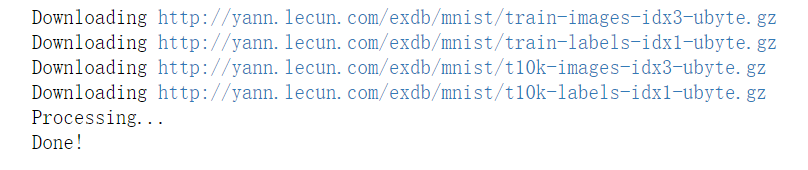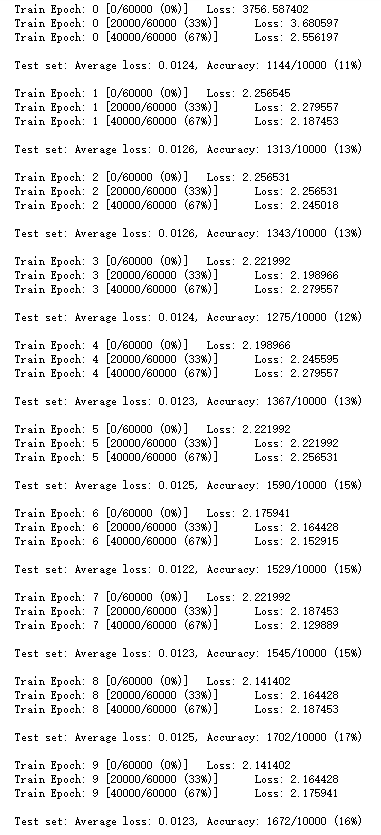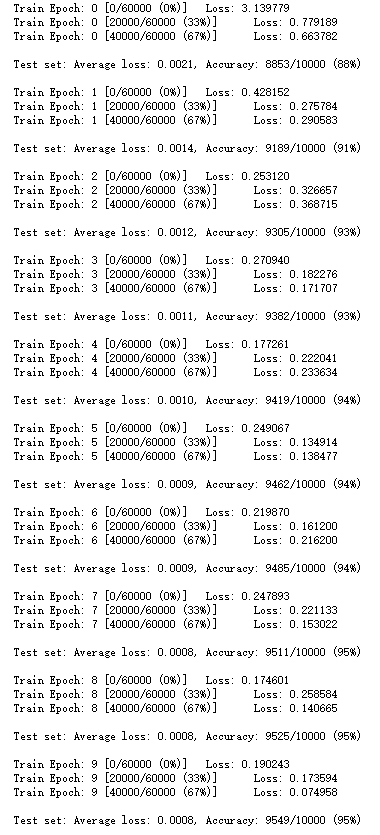# PyTorch做神经网络MINIST数据集多分类

PyTorch是Facebook的AI研究团队发布的python工具包，主要用于深度学习。这篇文章我们一起学习一下如何使用PyTorch搭建神经网络训练分类模型，这里我们用的数据集是Yann LeCun的MINIST数据集。首先我们来看看需要哪些python库：

import torch
import torch.nn as nn
import torch.nn.functional as F
import torch.optim as optim
from torchvision import datasets, transforms


batch_size = 200
learning_rate = 0.01
epochs = 10


train_loader = torch.utils.data.DataLoader(
datasets.MNIST('../data', train=True, download=True,
transform=transforms.Compose([
transforms.ToTensor(),
transforms.Normalize((0.1307,), (0.3081,))
])),
batch_size=batch_size, shuffle=True)

test_loader = torch.utils.data.DataLoader(
datasets.MNIST('../data', train=False, transform=transforms.Compose([
transforms.ToTensor(),
transforms.Normalize((0.1307,), (0.3081,))
])),
batch_size=batch_size, shuffle=True)w1, b1 = torch.randn(200, 784, requires_grad=True), torch.zeros(200, requires_grad=True)
w2, b2 = torch.randn(200, 200, requires_grad=True), torch.zeros(200, requires_grad=True)
w3, b3 = torch.randn(10, 200, requires_grad=True), torch.zeros(10, requires_grad=True)


def forward(x):
x = x@w1.t() + b1
x = F.relu(x)
x = x@w2.t() + b2
x = F.relu(x)
x = x@w3.t() + b3
x = F.relu(x)
return x


optimizer = optim.SGD([w1, b1, w2, b2, w3, b3], lr=learning_rate)
criteon = nn.CrossEntropyLoss()


SGD是常见的优化算法，里面传入的是模型的参数和学习率，然后算是函数是交叉熵。在所有的初始化完成之后，我们就可以进行模型训练了：

for epoch in range(epochs):

for batch_idx, (data, target) in enumerate(train_loader):
data = data.view(-1, 28*28)

logits = forward(data)
loss = criteon(logits, target)

optimizer.zero_grad()
loss.backward()
# print(w1.grad.norm(), w2.grad.norm())
optimizer.step()

if batch_idx % 100 == 0:
print('Train Epoch: {} [{}/{} ({:.0f}%)]\tLoss: {:.6f}'.format(
epoch, batch_idx * len(data), len(train_loader.dataset),
100. * batch_idx / len(train_loader), loss.item()))

test_loss = 0
correct = 0
for data, target in test_loader:
data = data.view(-1, 28 * 28)
logits = forward(data)
test_loss += criteon(logits, target).item()

pred = logits.data.max(1)
correct += pred.eq(target.data).sum()

test_loss /= len(test_loader.dataset)
print('\nTest set: Average loss: {:.4f}, Accuracy: {}/{} ({:.0f}%)\n'.format(
test_loss, correct, len(test_loader.dataset),
100. * correct / len(test_loader.dataset)))torch.nn.init.kaiming_normal_(w1)
torch.nn.init.kaiming_normal_(w2)
torch.nn.init.kaiming_normal_(w3)